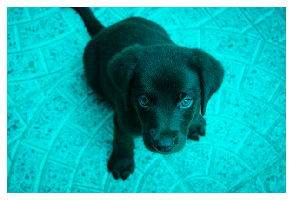# Implement new image transforms in fastai2

Hi!

I am trying to implement `rgb_randomize` in fastai2 following Sylvain suggestion. This is my first try to do something like this so I am a bit lost. I am trying to reuse some of the code from fastai1, which is:

``````def _rgb_randomize(x, channel:int=None, thresh:float=0.3):
"Randomize one of the channels of the input image"
if channel is None: channel = np.random.randint(0, x.shape - 1)
x[channel] = torch.rand(x.shape[1:]) * np.random.uniform(0, thresh)
return x

rgb_randomize = TfmPixel(_rgb_randomize)

class TfmPixel(Transform):
"Decorator for pixel tfm funcs."
order,_wrap = 10,'pixel'
``````

However, fastai1 and fastai2 implementations are quite different (at least for me). As I understand from the fastai2 09_vision.augment notebook, the structure to implement it should be something like:

1. Define class:
``````class _rgb_transform():
def __init__(self, channel:int=None, thresh:float=0.3):
store_attr(self, 'channel,thresh')
def before_call(self, x):
[...]
def __call__(self, x): return [...]

``````
1. Add it to `lighting` class?
``````@delegates(_rgb_transform.__init__)
@patch
def rgb_transform(x: TensorImage, **kwargs):
func = _rgb_transform(**kwargs)
func.before_call(x)
return x.lighting(func)
``````
1. Define `rgb_randomize` function
``````def rgb_randomize(x, channel:int=None, thresh:float=0.3):
"Randomize one of the channels of the input image"
return LightingTfm(_rgb_transform(channel,thresh))
``````

Is my understanding correct? I am sure that this could be wrong in a lot of ways. Any advice that shed some light on it would be very welcome.

2 Likes

Your transform is just applied on pixels, so it’s a regular function. Just create a subclass of `Transform` and defined in encodes the behavior you want (for `TensorImage` only, so make sure to have that type annotation).

Hi @sgugger,

Thanks for the feedback. I tried to build the function subclassing from `RandTransform` instead of `Tranform` in order to add a `p` value. However, the code:

``````def rgb_randomize(x:TensorImage, channel:int=None, thresh:float=0.3):
"Randomize one of the channels of the input image"
if channel is None: channel = np.random.randint(0, x.shape - 1)
x[channel] = torch.rand(x.shape[1:]) * np.random.uniform(0, thresh)
return x
``````
``````class rgb_transform(RandTransform):
def __init__(self, channel=None, thresh=0.3, p=0.5):
super().__init__(p=p)
self.channel,self.thresh,self.p = channel,thresh,p
def encodes(self, x:TensorImage): return x.rgb_randomize(channel=self.channel,thresh=self.thresh, p=self.p )
``````

Does not seems to apply any change in the example. I am not quite sure where the problem is but could be maybe because of `channel`? I see that if I specify `channel=4` or above does not throw any dimension error…

Any hints?
Thanks!

You need to pass `split_idx=0` when you call your transform to make it behave as if on the training set (otherwise, data aug is not applied). This is because You inherited from RandTransform.

Sorry for the misunderstanding, I already did it using:

``````_,axs = subplots(2, 4)
for ax in axs.flatten():
show_image(rgb_transform(channel=1, thresh=0.99, p=1.)(img, split_idx=0), ctx=ax)
``````

Also, subclassing from `Transform` gives the same result. I used:

``````class rgb_transform(Transform):
def __init__(self, channel=None, thresh=0.3):
self.channel,self.thresh = channel,thresh
def encodes(self, x:TensorImage): return x.rgb_randomize(channel=self.channel,thresh=self.thresh)
``````

But I am always getting the source image without any change.

EDIT

It seems that the indexing was not properly done:

If I do `x = TensorImage(img)` and then `x.shape` I got `torch.Size([600, 3])` so I was indexing in the wrong dimension. If I modify and do:

``````channel = 1
x = TensorImage(img)
x[:,:,channel] = torch.rand(x[:,:,channel].shape) * np.random.uniform(0.1, 0.3)
show_image(x)
``````

I got more or less the expected:
`show_image(x)`However, I have still two issues. First, `x[:,:,channel] = torch.rand(x[:,:,channel].shape) * np.random.uniform(0.1, 0.3); x[:,:,channel]` gives always

``````tensor([[0, 0, 0,  ..., 0, 0, 0],
[0, 0, 0,  ..., 0, 0, 0],
[0, 0, 0,  ..., 0, 0, 0],
...,
[0, 0, 0,  ..., 0, 0, 0],
[0, 0, 0,  ..., 0, 0, 0],
[0, 0, 0,  ..., 0, 0, 0]], dtype=torch.uint8)
``````

but printing `torch.rand(x[:,:,channel].shape) * np.random.uniform(0.1, 0.3)` gives proper output

``````tensor([[0.1735, 0.0108, 0.2265,  ..., 0.2204, 0.1518, 0.0196],
[0.0779, 0.1818, 0.0029,  ..., 0.0849, 0.0894, 0.0246],
[0.0235, 0.0237, 0.1356,  ..., 0.1863, 0.0127, 0.0546],
...,
[0.1230, 0.2265, 0.0699,  ..., 0.0486, 0.2083, 0.1266],
[0.0639, 0.1129, 0.2149,  ..., 0.1958, 0.1331, 0.1243],
[0.1772, 0.0370, 0.0839,  ..., 0.2271, 0.1627, 0.0231]])
``````

Second, when perform the changes in the class the output is still the same as the source. The code:

``````def rgb_randomize(x:TensorImage, channel:int=None, thresh:float=0.3):
"Randomize one of the channels of the input image"
if channel is None: channel = np.random.randint(0, x.shape - 1)
x[:,:,channel] = torch.rand(x[:,:,channel].shape) * np.random.uniform(0, thresh)
return x
class rgb_transform(RandTransform):
def __init__(self, channel=None, thresh=0.3, p=0.5):
super().__init__(p=p)
self.channel,self.thresh,self.p = channel,thresh,p
def encodes(self, x:TensorImage): return x.rgb_randomize(channel=self.channel,thresh=self.thresh, p=self.p )
_,axs = subplots(2, 4)
for ax in axs.flatten():
show_image(rgb_transform(channel=0, thresh=0.3, p=1)(img, split_idx=0), ctx=ax)
``````

EDIT2 ping @sgugger. Sorry to bother you again, I am not able to see where the problem isYour code does not have the patch decorator, so I don’t how `x.rgb_randomize` could work.